# Introduction

The FLAME framework is an enabling tool to create agent-based models that can be run on high performance computers (HPCs). Models are created based upon a model of computation called (extended finite) state machines. By defining agent-based models in this way the FLAME framework can automatically generate simulation programs that can run models efficiently on HPCs.

# Model Design

The philosophy of FLAME is to specify an agent-based model as you would specify software behaviour, as ultimately the execution of the model will be in software. The behaviour model is based upon state machines which are composed of a number of states with transition functions between those states. There is a single start state and by traversing states using the transition functions the machine executes the functions until it reaches an end state. This happens to each agent/machine as one time step or iteration is completed. Figure 1 shows a model consisting of two agents each with two functions run one after the other. A time step or iteration of the model is when each agent goes from their start state to an end state.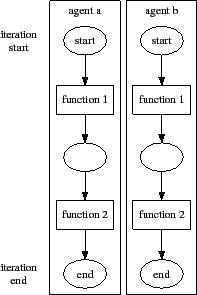Each agent has a memory that holds variables. Transition functions can read and write to variables in the agent's memory. Communication between agents is achieved via messages. Transition functions can also read incoming messages and write outgoing messages.

Because FLAME can execute agent models in parallel and on different processors the coordination of agent function execution depends on the communication between agents. Communication is synchronous to the agent model which means it happens at the same time. For agents this means that if an agent function receives a certain type of message, it cannot be executed until all the messages of that type have been sent and that they are all ready to be read. This means that no agent is given priority over reading any input and all agents have access to the same messages at the same time. This also means that the order that agents are executed does not matter.

Because agents can be executed anywhere on a super computer cluster FLAME uses a broadcast communication method. This means that agents cannot directly send messages to another agent. Instead the receiving agent must filter messages that it only needs to read.

Figure 2 shows an agent machine with a start state, an end state and one transition function from one to the other which has access to the agent memory and recieves input messages and produces output messages.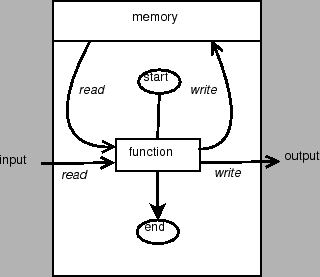Describing an agent-based model would thus include the following individual stages for creating a model:

• Identifying the agents and their functions
• Identify the states which impose some order of function execution
• Identify the memory as the set of variables that are accessed by functions
(including possible conditions on variables for the functions to occur)
• Identify the input messages and output messages of each function
(including possible filters on inputs)

Once a model has been defined using these criteria the implementation of the agent functions can be written as source code in the C programming language. FLAME can then use the model description to create a simulation program that handles agent execution and communication in parallel.

## Swarm Example

For example a simple swarm (flocking) model would include an agent for a bird. Because agents can only communicate via messages in FLAME each bird needs to have a function that sends out a message with their current location. A second function is needed to read the messages and update the birds velocity depending on the other birds locations. A third function then updates the birds location using the birds new velocity. The three functions required of the bird agent are then:

• signal - send out current position message
• observe - read position messages from other agents and update velocity
• respond - update position using the current velocity

The functions occur in this order so states are included to impose this order, see Figure 3. As a requirement for automatic parallel execution agents can only enter particular states once during an iteration, i.e. there cannot be any loops back to a state already entered. This is so that parallel processes can easily stay synchronised, adding to the efficiently of a simulation. There can only be one start state per agent, but there can be many possible end states.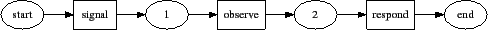Functions can also have conditions. For instance, in the swarm model, a response function for flying and a response function when resting on the ground. The condition on the flying response function would be that the z-axis position of the agent be greater than zero while the resting response function condition would be when the z-axis position was zero, see Figure 4.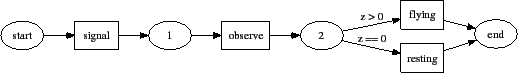The messages required for communication between agents are a signal message, which is output from `signal' and input to `observe', see Figure 5. This message would include the position of the agent that sent it, see Table 1. A feature of swarm models and most agent-based models is that there is generally a limit on incoming communication. In the swarm case this is the perceived distance of sight that an agent can view the location of other agents. This feature can be added to the model as a filter on inputs to a function, where the filter is a formula involving the position contained in the message (the position of the sending agent) and the receiving agent position.

Table 1: Signal Message
 Type Name Description double px x-axis position double py y-axis position double pz z-axis position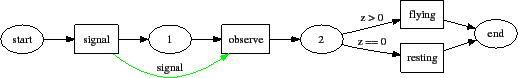Functions that take a message type as input are only executed once all functions that output the same message type have finished. One iteration is taken as a standalone run of a simulation, so once all the functions that have a message type as an input have been executed, the messages are deleted as they are no longer required. Messages cannot be sent between iterations.

Finally the memory required by the agent functions include the position of the agent, and its velocity, as shown in Table 2.

Table 2: Swarm Agent Memory
 Type Name Description double px position in x-axis double py position in y-axis double pz position in z-axis double vx velocity in x-axis double vy velocity in y-axis double vz velocity in z-axis

The swarm model can also be represented as a transition table, see Table 3, where:

• Current State - is the state the agent is currently in.
• Input - is any inputs into the transition function.
•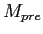- are any preconditions of the memory on the transition.
• Function - is the function name.
•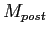- is any change in the agent memory.
• Output - is any outputs from the transition.
• Next State - is the next state that is entered by the agent.

Table 3: Swarm Agent Transition Table
 Current State InputFunctionOutput Next State start signal signal 1 1 signal observe (velocity updated) 2 2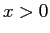flying (position updated) end 2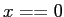resting (position updated) end